# CS 558 COMPUTER VISION Lecture IV Image Filter

• Slides: 79
Download presentationCS 558 COMPUTER VISION Lecture IV: Image Filter and Edge Detection Slides adapted from S. Lazebnik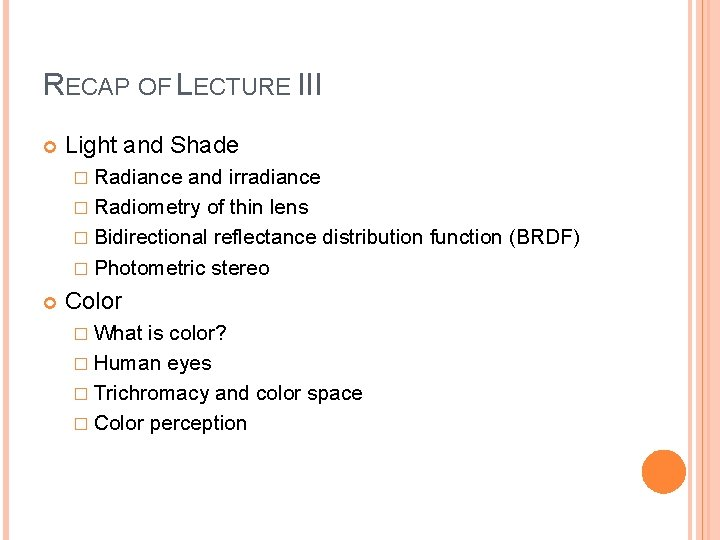RECAP OF LECTURE III Light and Shade � Radiance and irradiance � Radiometry of thin lens � Bidirectional reflectance distribution function (BRDF) � Photometric stereo Color � What is color? � Human eyes � Trichromacy and color space � Color perception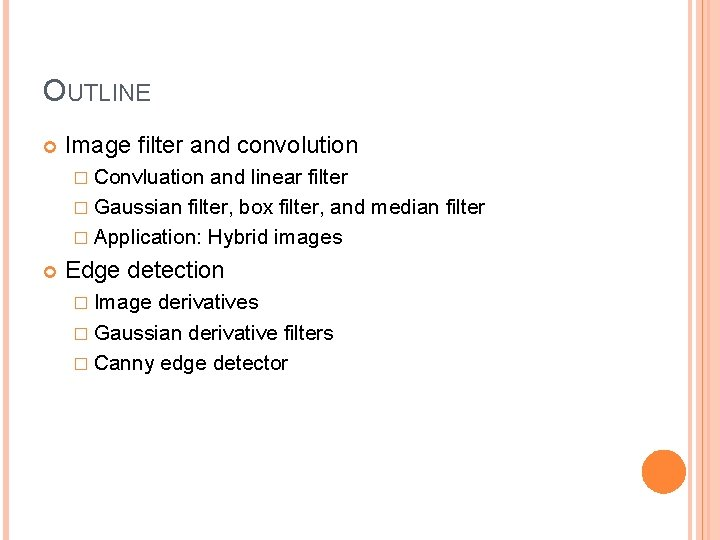OUTLINE Image filter and convolution � Convluation and linear filter � Gaussian filter, box filter, and median filter � Application: Hybrid images Edge detection � Image derivatives � Gaussian derivative filters � Canny edge detector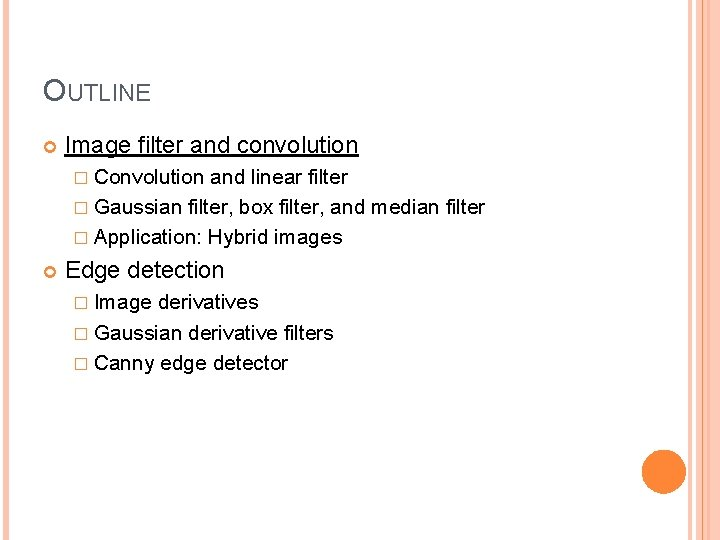OUTLINE Image filter and convolution � Convolution and linear filter � Gaussian filter, box filter, and median filter � Application: Hybrid images Edge detection � Image derivatives � Gaussian derivative filters � Canny edge detector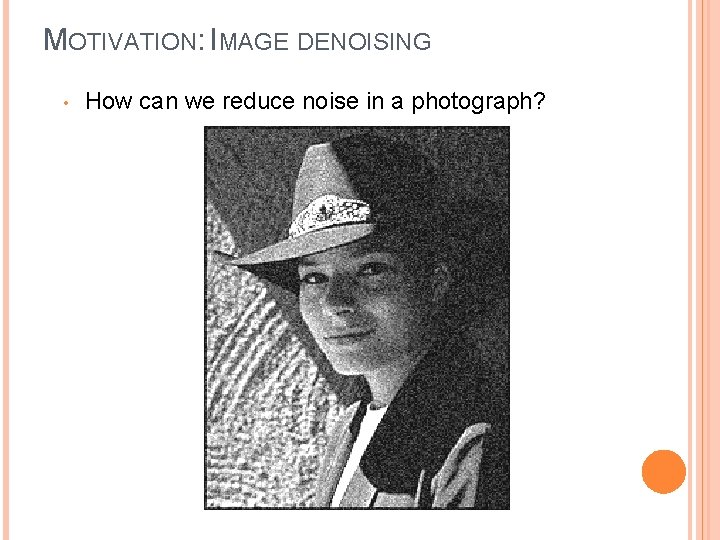MOTIVATION: IMAGE DENOISING • How can we reduce noise in a photograph?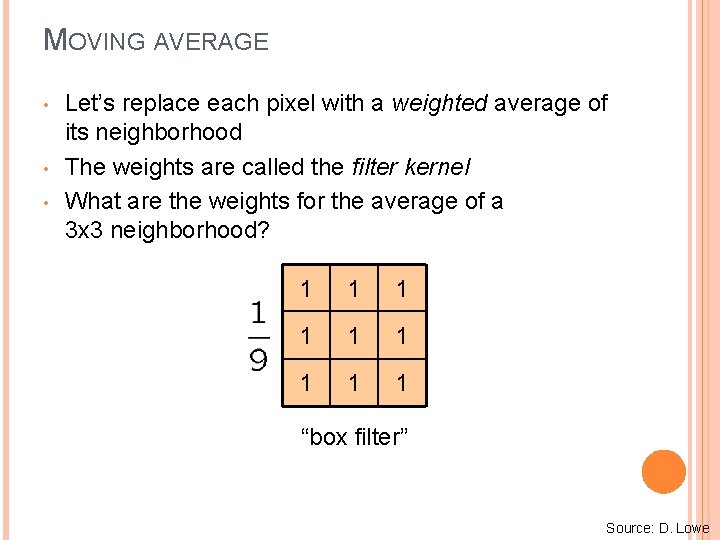MOVING AVERAGE • • • Let’s replace each pixel with a weighted average of its neighborhood The weights are called the filter kernel What are the weights for the average of a 3 x 3 neighborhood? 1 1 1 1 1 “box filter” Source: D. Lowe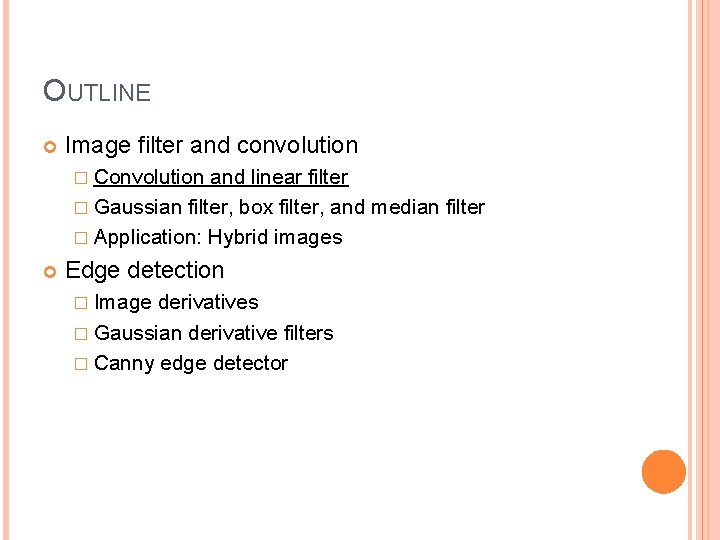OUTLINE Image filter and convolution � Convolution and linear filter � Gaussian filter, box filter, and median filter � Application: Hybrid images Edge detection � Image derivatives � Gaussian derivative filters � Canny edge detectorDEFINING CONVOLUTION • Let f be the image and g be the kernel. The output of convolving f with g is denoted f *g. f • MATLAB functions: conv 2, filter 2, imfilter Source: F. Durand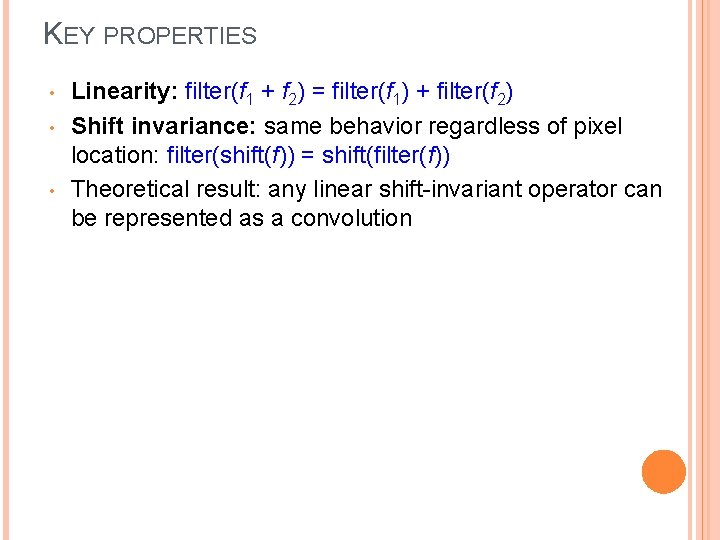KEY PROPERTIES • • • Linearity: filter(f 1 + f 2) = filter(f 1) + filter(f 2) Shift invariance: same behavior regardless of pixel location: filter(shift(f)) = shift(filter(f)) Theoretical result: any linear shift-invariant operator can be represented as a convolution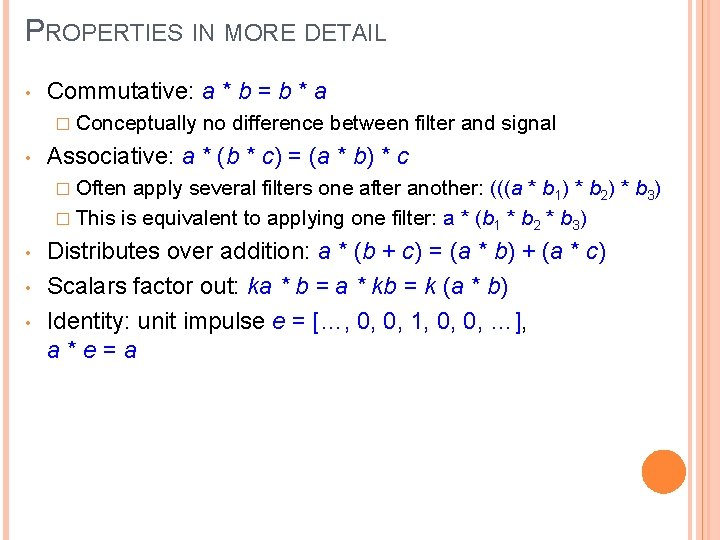PROPERTIES IN MORE DETAIL • Commutative: a * b = b * a � Conceptually • no difference between filter and signal Associative: a * (b * c) = (a * b) * c � Often apply several filters one after another: (((a * b 1) * b 2) * b 3) � This is equivalent to applying one filter: a * (b 1 * b 2 * b 3) • • • Distributes over addition: a * (b + c) = (a * b) + (a * c) Scalars factor out: ka * b = a * kb = k (a * b) Identity: unit impulse e = […, 0, 0, 1, 0, 0, …], a*e=aANNOYING DETAILS • What is the size of the output? MATLAB: filter 2(g, f, shape) � shape = ‘full’: output size is sum of sizes of f and g � shape = ‘same’: output size is same as f � shape = ‘valid’: output size is difference of sizes of f and g full g g same g f g valid g g f g g gANNOYING DETAILS What about near the edge? � the filter window falls off the edge of the image � need to extrapolate � methods: clip filter (black) wrap around copy edge reflect across edge Source: S. MarschnerANNOYING DETAILS What about near the edge? � the filter window falls off the edge of the image � need to extrapolate � methods (MATLAB): clip filter (black): imfilter(f, g, 0) wrap around: imfilter(f, g, ‘circular’) copy edge: imfilter(f, g, ‘replicate’) reflect across edge: imfilter(f, g, ‘symmetric’) Source: S. MarschnerPRACTICE WITH LINEAR FILTERS 0 0 1 0 0 ? Original Source: D. LowePRACTICE WITH LINEAR FILTERS 0 0 1 0 0 Original Filtered (no change) Source: D. LowePRACTICE WITH LINEAR FILTERS 0 0 0 1 0 0 0 ? Original Source: D. LowePRACTICE WITH LINEAR FILTERS 0 0 0 1 0 0 0 Original Shifted left By 1 pixel Source: D. Lowe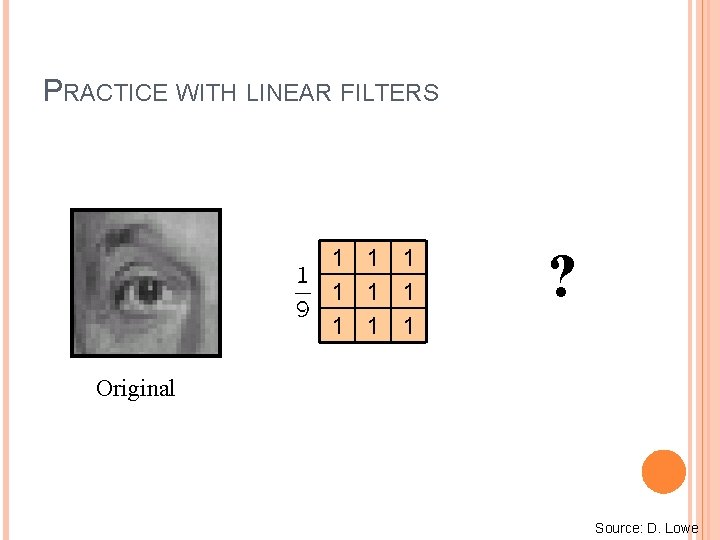PRACTICE WITH LINEAR FILTERS 1 1 1 1 1 ? Original Source: D. Lowe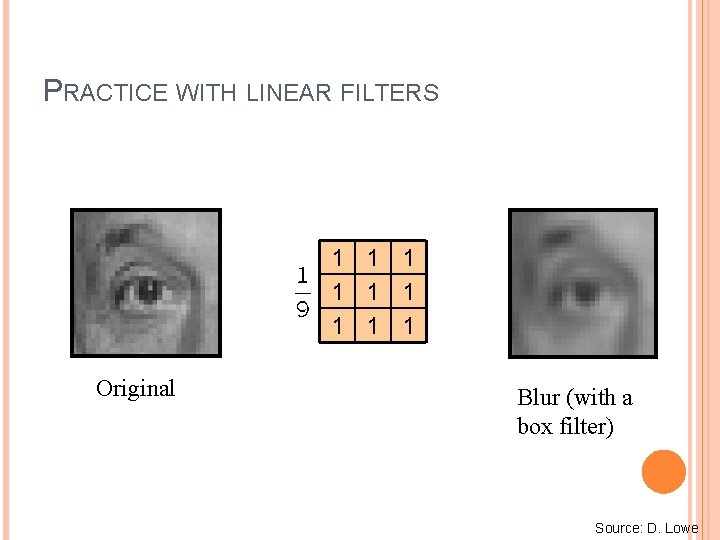PRACTICE WITH LINEAR FILTERS 1 1 1 1 1 Original Blur (with a box filter) Source: D. Lowe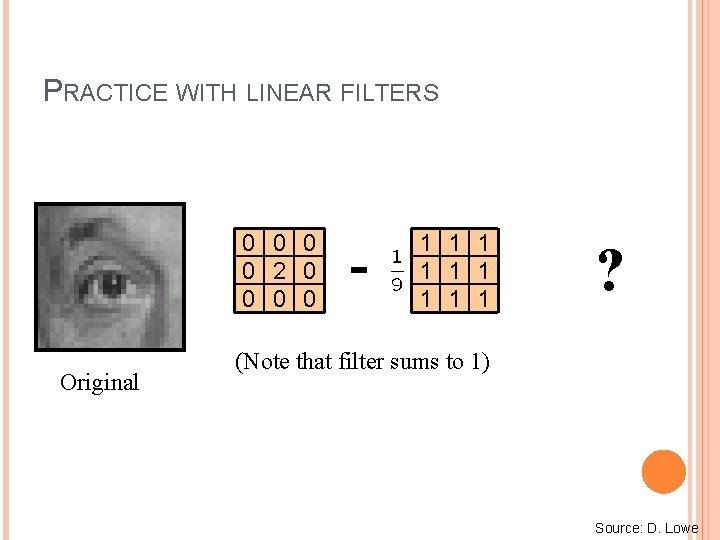PRACTICE WITH LINEAR FILTERS 0 0 2 0 0 Original - 1 1 1 1 1 ? (Note that filter sums to 1) Source: D. LowePRACTICE WITH LINEAR FILTERS 0 0 2 0 0 Original - 1 1 1 1 1 Sharpening filter - Accentuates differences with local average Source: D. LoweSHARPENING Source: D. Lowe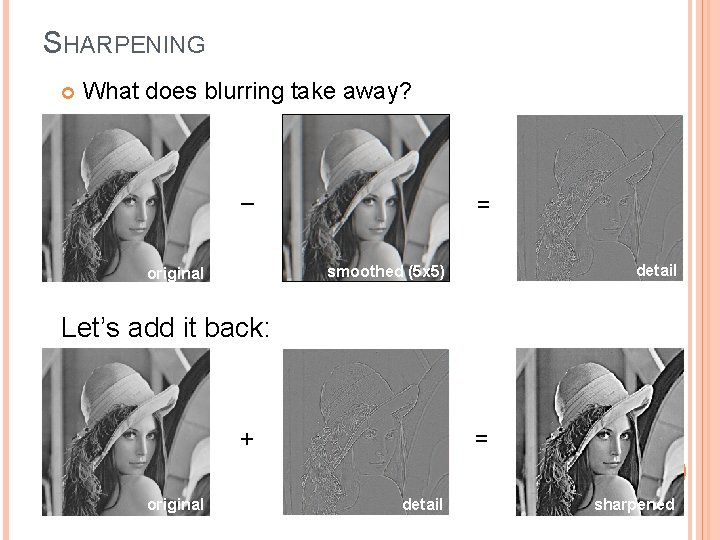SHARPENING What does blurring take away? – = detail smoothed (5 x 5) original Let’s add it back: + original = detail sharpened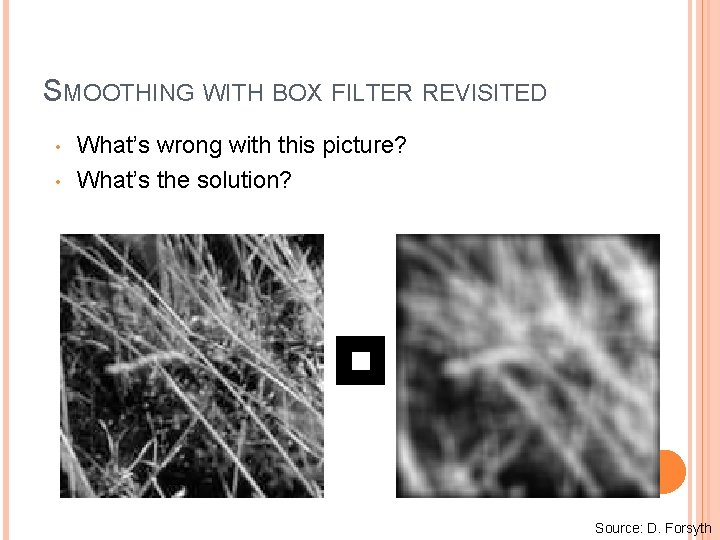SMOOTHING WITH BOX FILTER REVISITED • • What’s wrong with this picture? What’s the solution? Source: D. ForsythSMOOTHING WITH BOX FILTER REVISITED • • What’s wrong with this picture? What’s the solution? � To eliminate edge effects, weight contribution of neighborhood pixels according to their closeness to the center “fuzzy blob”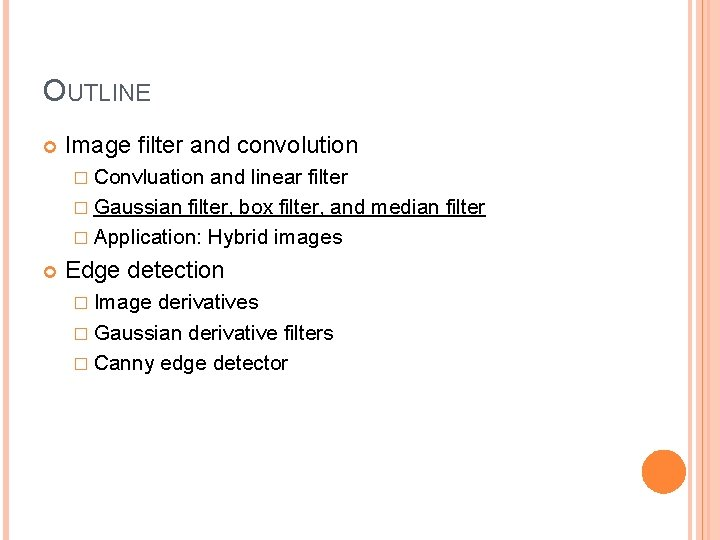OUTLINE Image filter and convolution � Convluation and linear filter � Gaussian filter, box filter, and median filter � Application: Hybrid images Edge detection � Image derivatives � Gaussian derivative filters � Canny edge detector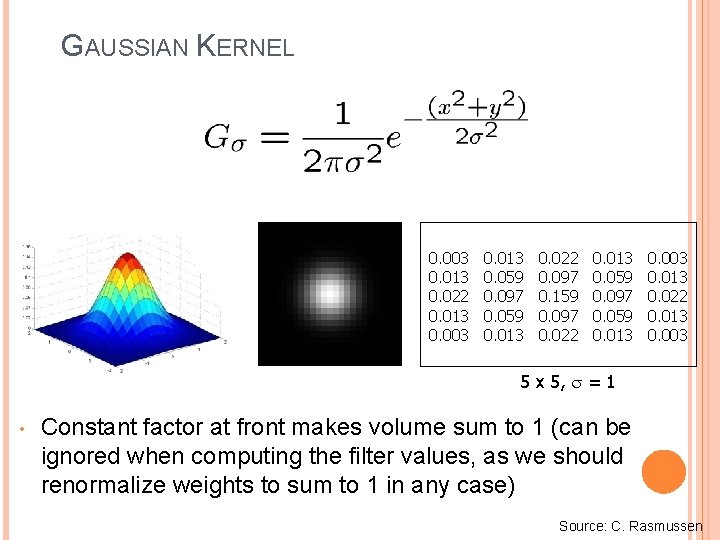GAUSSIAN KERNEL 0. 003 0. 013 0. 022 0. 013 0. 003 0. 013 0. 059 0. 097 0. 059 0. 013 0. 022 0. 097 0. 159 0. 097 0. 022 0. 013 0. 059 0. 097 0. 059 0. 013 0. 003 0. 013 0. 022 0. 013 0. 003 5 x 5, = 1 • Constant factor at front makes volume sum to 1 (can be ignored when computing the filter values, as we should renormalize weights to sum to 1 in any case) Source: C. Rasmussen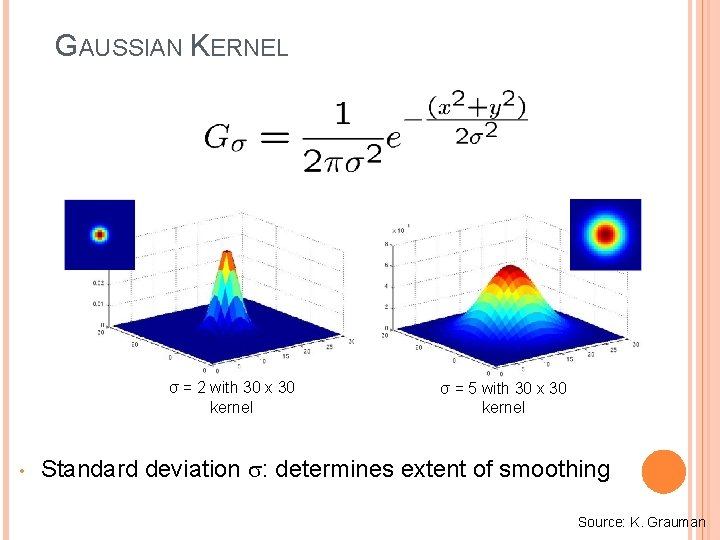GAUSSIAN KERNEL σ = 2 with 30 x 30 kernel • σ = 5 with 30 x 30 kernel Standard deviation : determines extent of smoothing Source: K. Grauman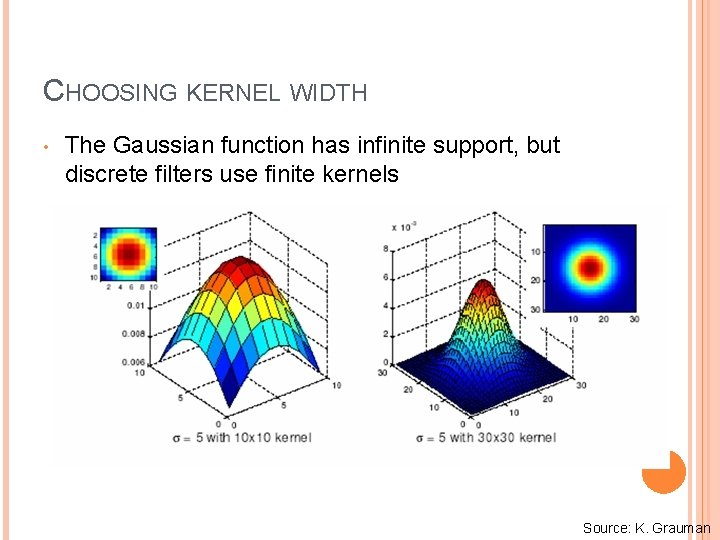CHOOSING KERNEL WIDTH • The Gaussian function has infinite support, but discrete filters use finite kernels Source: K. GraumanCHOOSING KERNEL WIDTH • Rule of thumb: set filter half-width to about 3σGAUSSIAN VS. BOX FILTERING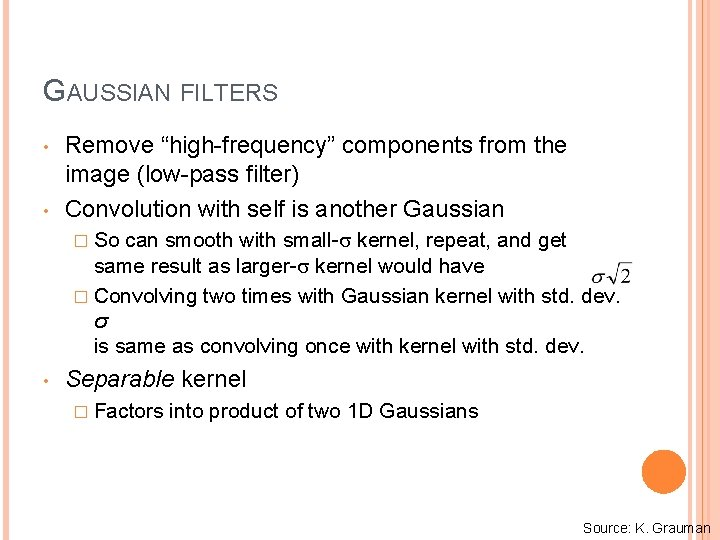GAUSSIAN FILTERS • • Remove “high-frequency” components from the image (low-pass filter) Convolution with self is another Gaussian � So can smooth with small- kernel, repeat, and get same result as larger- kernel would have � Convolving two times with Gaussian kernel with std. dev. σ is same as convolving once with kernel with std. dev. • Separable kernel � Factors into product of two 1 D Gaussians Source: K. GraumanSEPARABILITY OF THE GAUSSIAN FILTER Source: D. LoweSEPARABILITY EXAMPLE 2 D convolution (center location only) The filter factors into a product of 1 D filters: Perform convolution along rows: * = Followed by convolution along the remaining column: * = Source: K. Grauman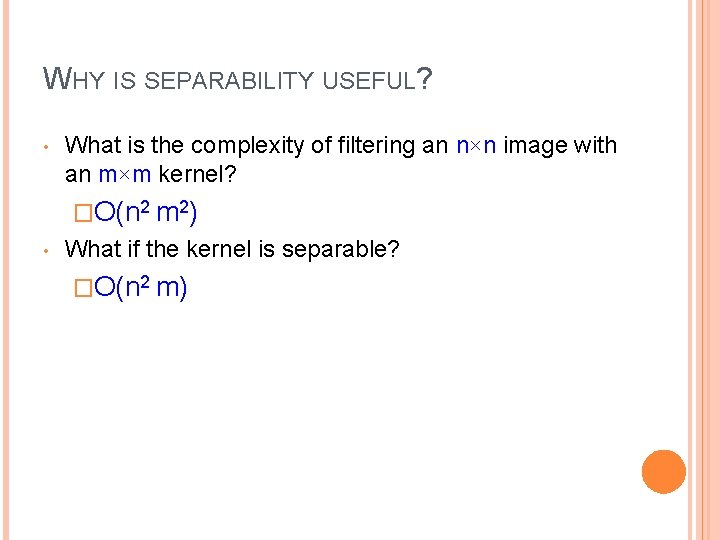WHY IS SEPARABILITY USEFUL? • What is the complexity of filtering an n×n image with an m×m kernel? �O(n 2 m 2) • What if the kernel is separable? �O(n 2 m)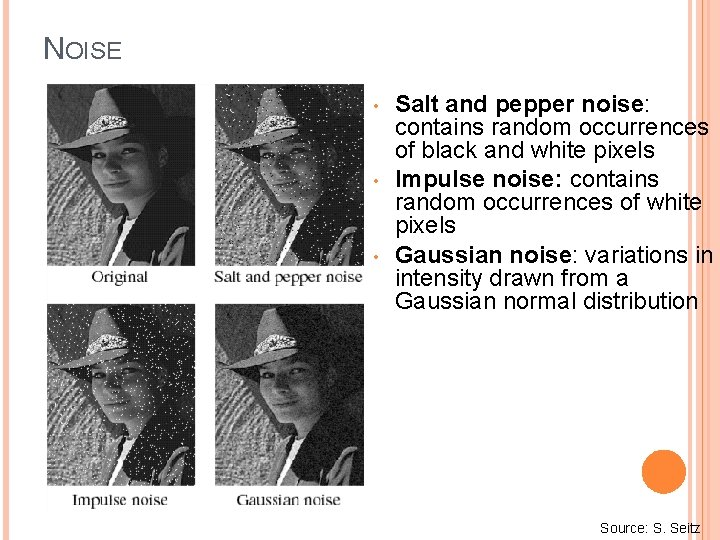NOISE • • • Salt and pepper noise: contains random occurrences of black and white pixels Impulse noise: contains random occurrences of white pixels Gaussian noise: variations in intensity drawn from a Gaussian normal distribution Source: S. Seitz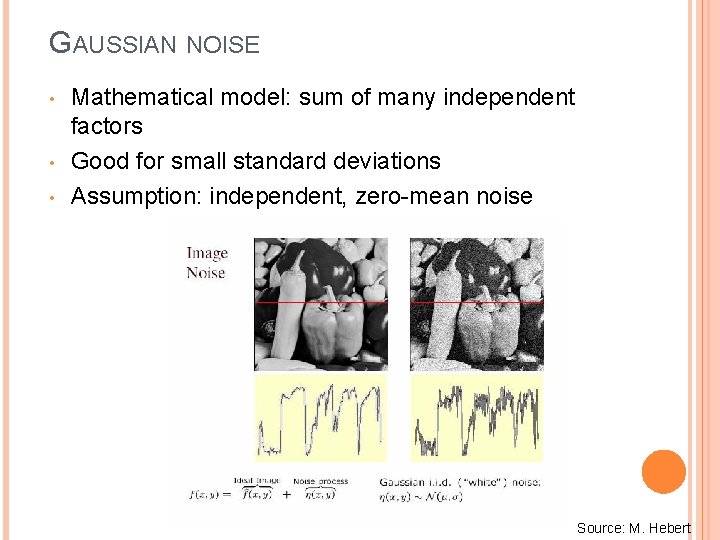GAUSSIAN NOISE • • • Mathematical model: sum of many independent factors Good for small standard deviations Assumption: independent, zero-mean noise Source: M. Hebert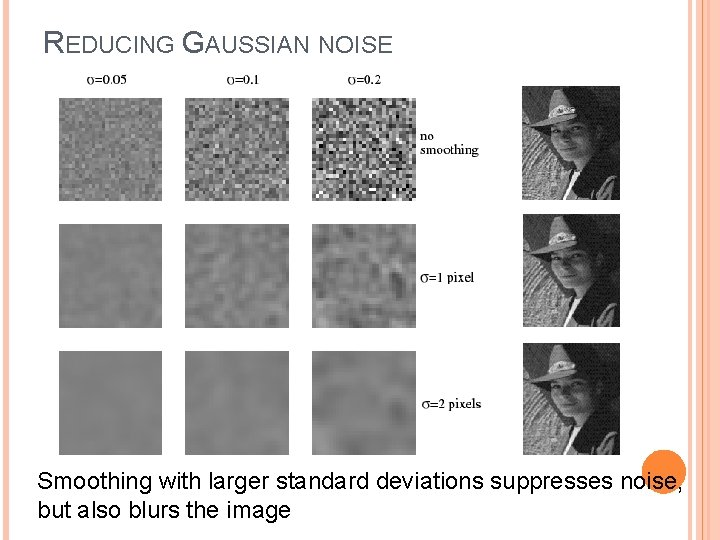REDUCING GAUSSIAN NOISE Smoothing with larger standard deviations suppresses noise, but also blurs the imageREDUCING SALT-AND-PEPPER NOISE 3 x 3 5 x 5 What’s wrong with the results? 7 x 7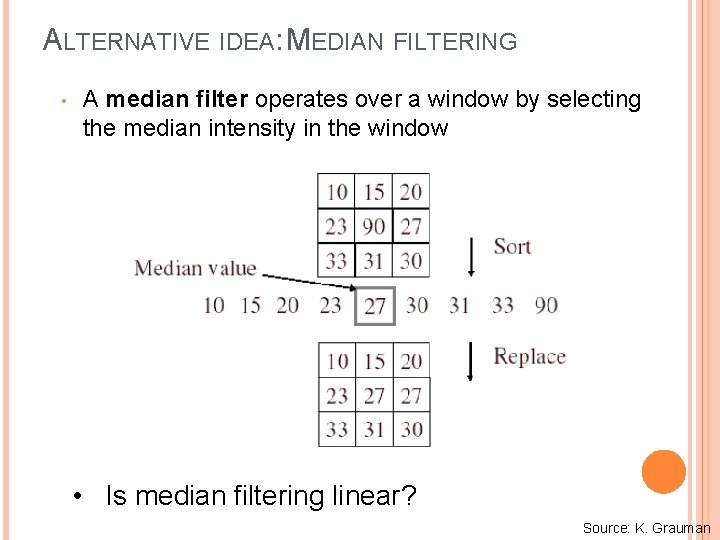ALTERNATIVE IDEA: MEDIAN FILTERING • A median filter operates over a window by selecting the median intensity in the window • Is median filtering linear? Source: K. Grauman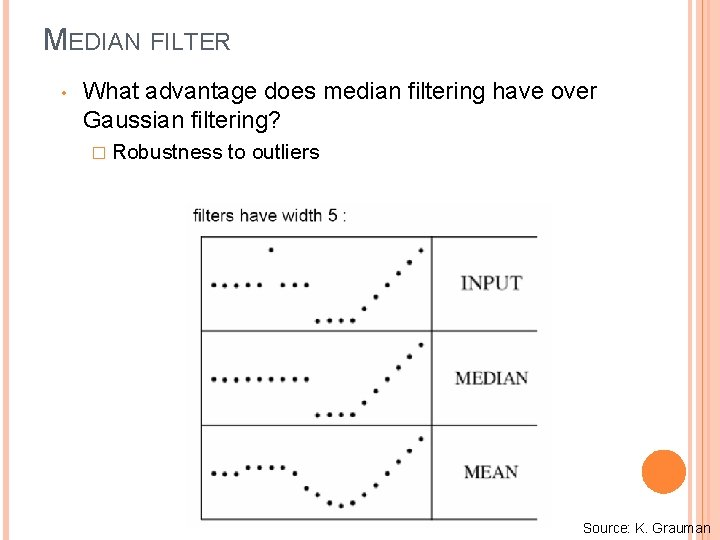MEDIAN FILTER • What advantage does median filtering have over Gaussian filtering? � Robustness to outliers Source: K. GraumanMEDIAN FILTER Salt-and-pepper noise Median filtered MATLAB: medfilt 2(image, [h w]) Source: M. HebertGAUSSIAN VS. MEDIAN FILTERING 3 x 3 Gaussian Median 5 x 5 7 x 7SHARPENING REVISITED Source: D. LoweSHARPENING REVISITED What does blurring take away? – = detail smoothed (5 x 5) original Let’s add it back: +α original = detail sharpenedUNSHARP MASK FILTER image unit impulse (identity) blurred image Gaussian Laplacian of GaussianOUTLINE Image filter and convolution � Convolution and linear filter � Gaussian filter, box filter, and median filter � Application: Hybrid images Edge detection � Image derivatives � Gaussian derivative filters � Canny edge detector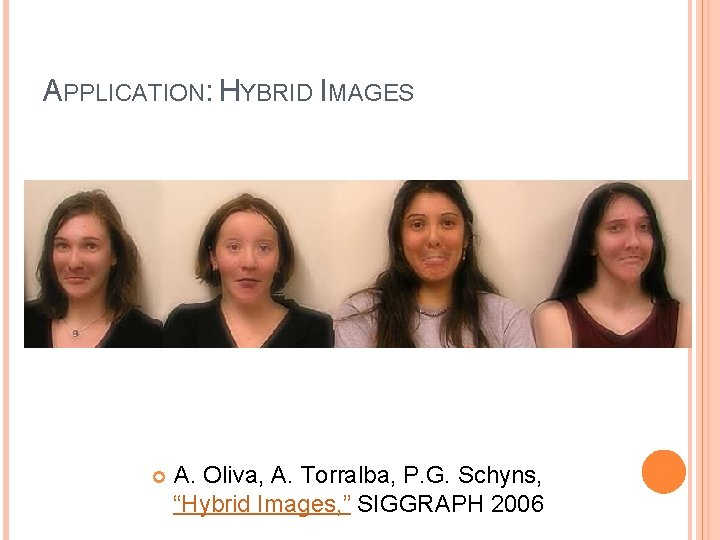APPLICATION: HYBRID IMAGES A. Oliva, A. Torralba, P. G. Schyns, “Hybrid Images, ” SIGGRAPH 2006APPLICATION: HYBRID IMAGES Gaussian Filter Laplacian Filter A. Oliva, A. Torralba, P. G. Schyns, “Hybrid Images, ” SIGGRAPH 2006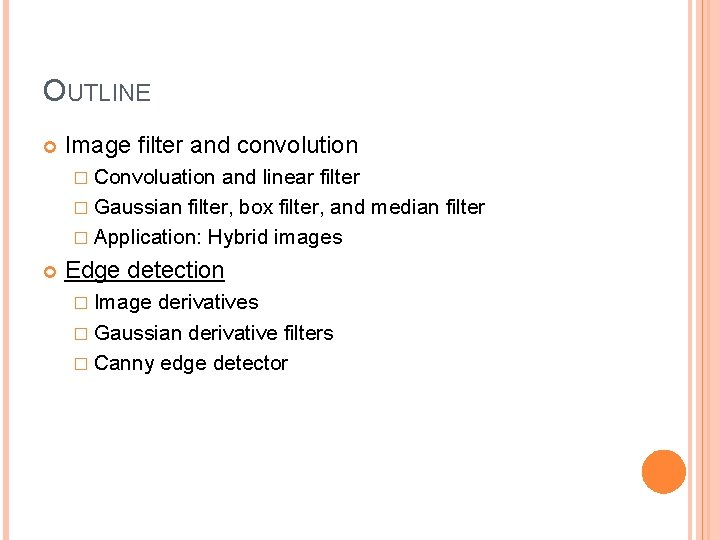OUTLINE Image filter and convolution � Convoluation and linear filter � Gaussian filter, box filter, and median filter � Application: Hybrid images Edge detection � Image derivatives � Gaussian derivative filters � Canny edge detector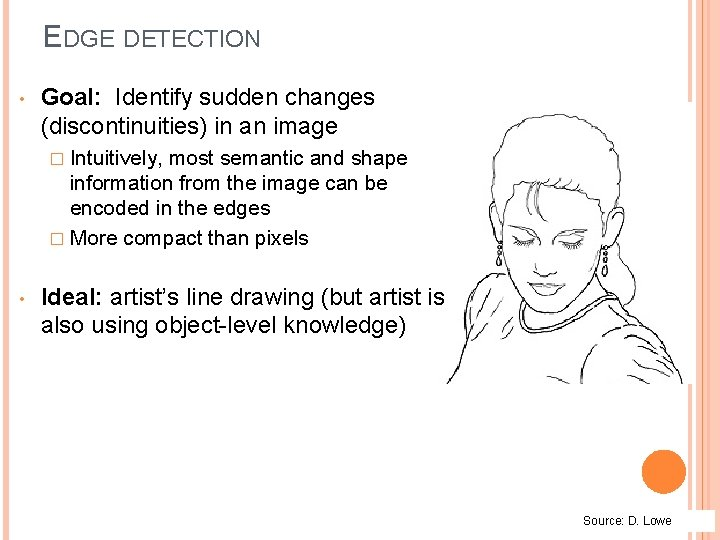EDGE DETECTION • Goal: Identify sudden changes (discontinuities) in an image � Intuitively, most semantic and shape information from the image can be encoded in the edges � More compact than pixels • Ideal: artist’s line drawing (but artist is also using object-level knowledge) Source: D. LoweORIGIN OF EDGES Edges are caused by a variety of factors: surface normal discontinuity depth discontinuity surface color discontinuity illumination discontinuity Source: Steve Seitz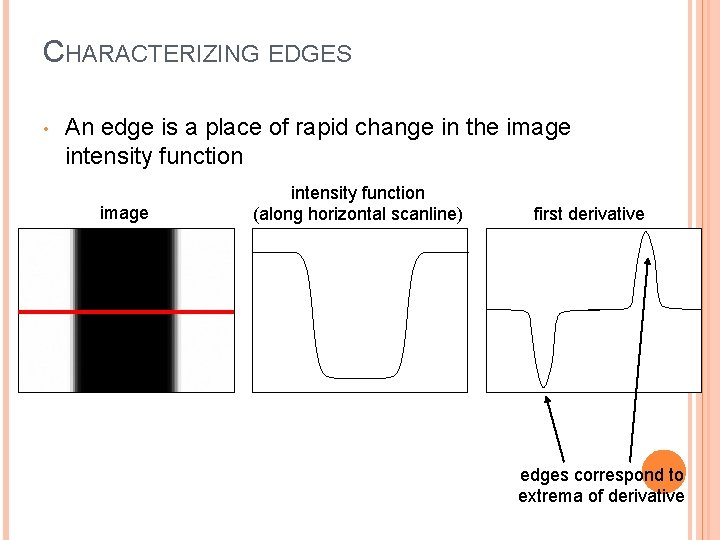CHARACTERIZING EDGES • An edge is a place of rapid change in the image intensity function (along horizontal scanline) first derivative edges correspond to extrema of derivative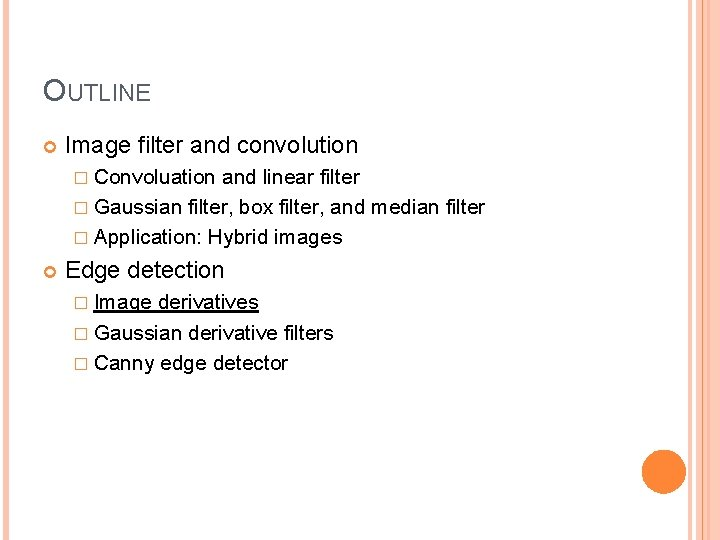OUTLINE Image filter and convolution � Convoluation and linear filter � Gaussian filter, box filter, and median filter � Application: Hybrid images Edge detection � Image derivatives � Gaussian derivative filters � Canny edge detector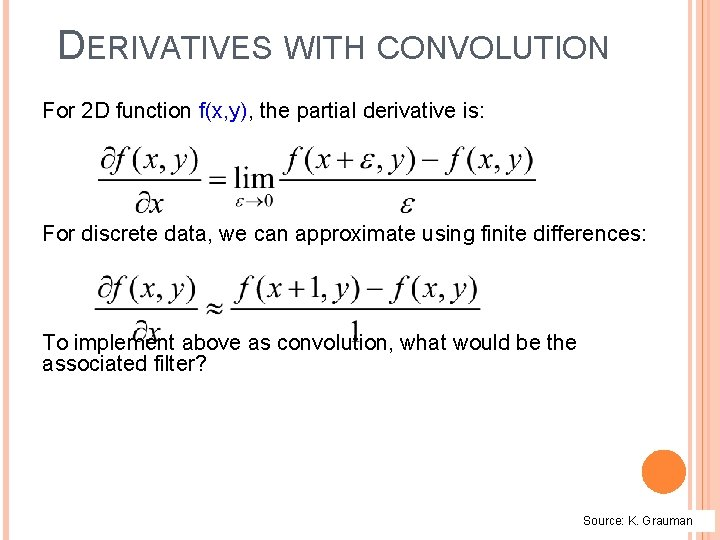DERIVATIVES WITH CONVOLUTION For 2 D function f(x, y), the partial derivative is: For discrete data, we can approximate using finite differences: To implement above as convolution, what would be the associated filter? Source: K. GraumanPARTIAL DERIVATIVES OF AN IMAGE -1 -1 1 1 Which shows changes with respect to x? or 1 -1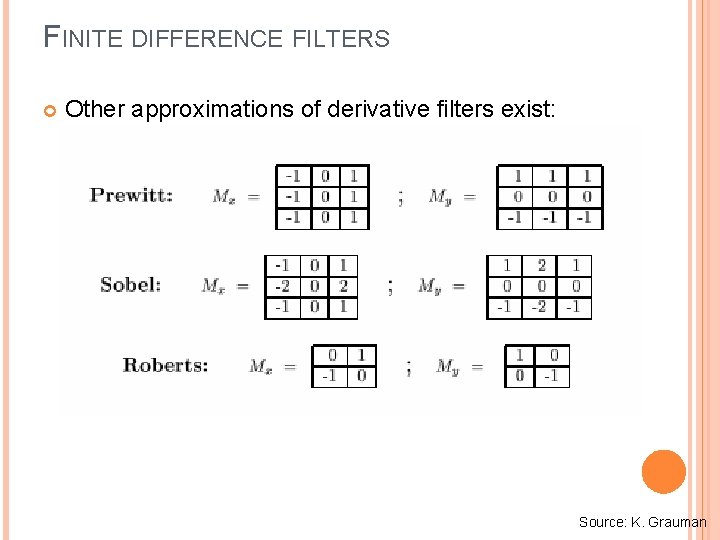FINITE DIFFERENCE FILTERS Other approximations of derivative filters exist: Source: K. Grauman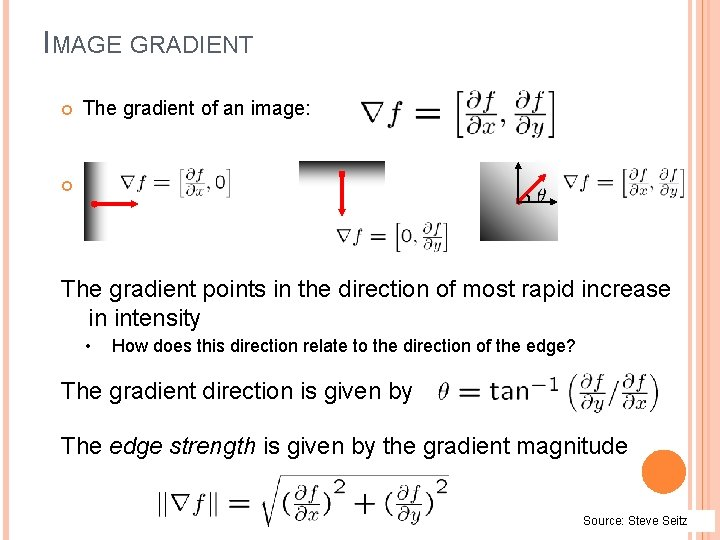IMAGE GRADIENT The gradient of an image: The gradient points in the direction of most rapid increase in intensity • How does this direction relate to the direction of the edge? The gradient direction is given by The edge strength is given by the gradient magnitude Source: Steve Seitz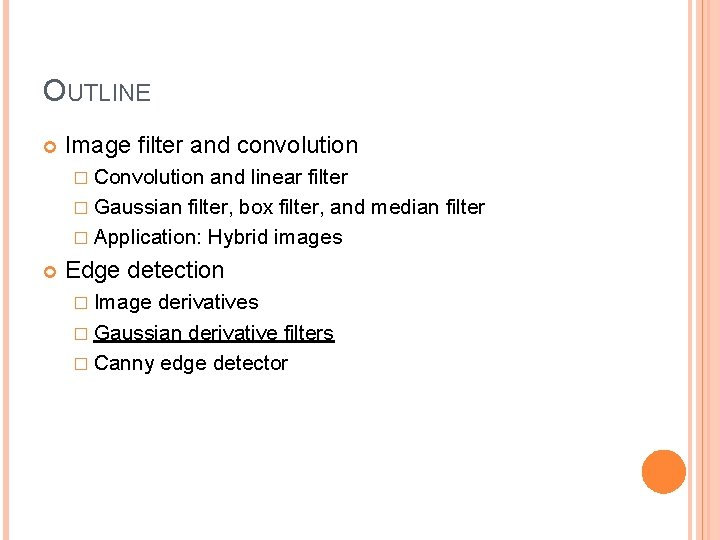OUTLINE Image filter and convolution � Convolution and linear filter � Gaussian filter, box filter, and median filter � Application: Hybrid images Edge detection � Image derivatives � Gaussian derivative filters � Canny edge detectorEFFECTS OF NOISE Consider a single row or column of the image � Plotting intensity as a function of position gives a signal Where is the edge? Source: S. Seitz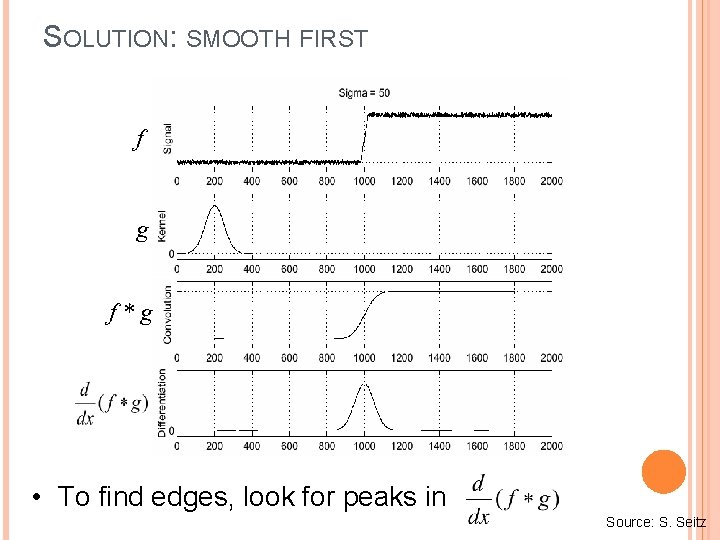SOLUTION: SMOOTH FIRST f g f*g • To find edges, look for peaks in Source: S. Seitz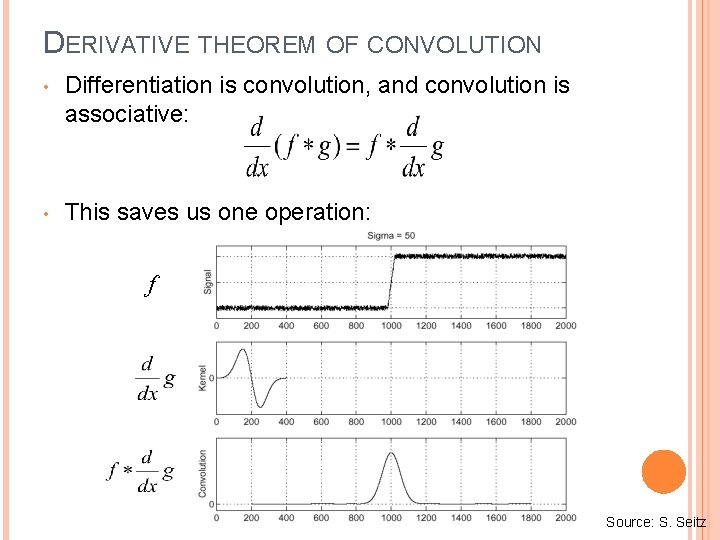DERIVATIVE THEOREM OF CONVOLUTION • Differentiation is convolution, and convolution is associative: • This saves us one operation: f Source: S. Seitz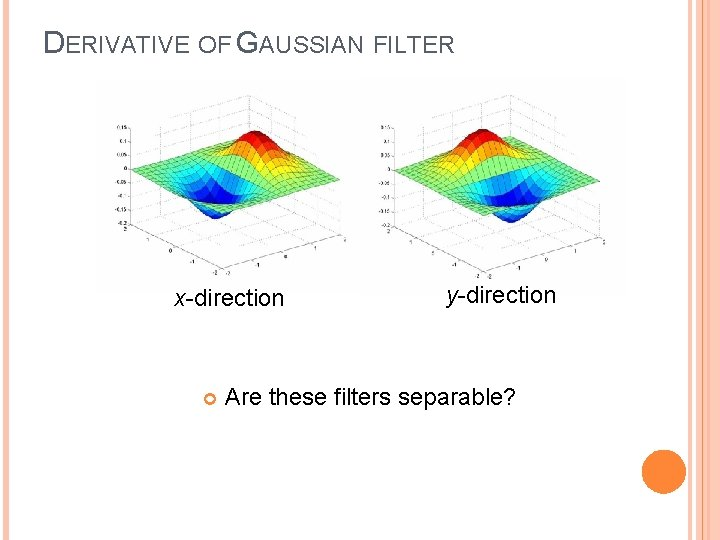DERIVATIVE OF GAUSSIAN FILTER x-direction y-direction Are these filters separable?DERIVATIVE OF GAUSSIAN FILTER x-direction y-direction Which one finds horizontal/vertical edges?SCALE OF GAUSSIAN DERIVATIVE FILTER 1 pixel 3 pixels 7 pixels Smoothed derivative removes noise, but blurs edge. Also finds edges at different “scales” Source: D. Forsyth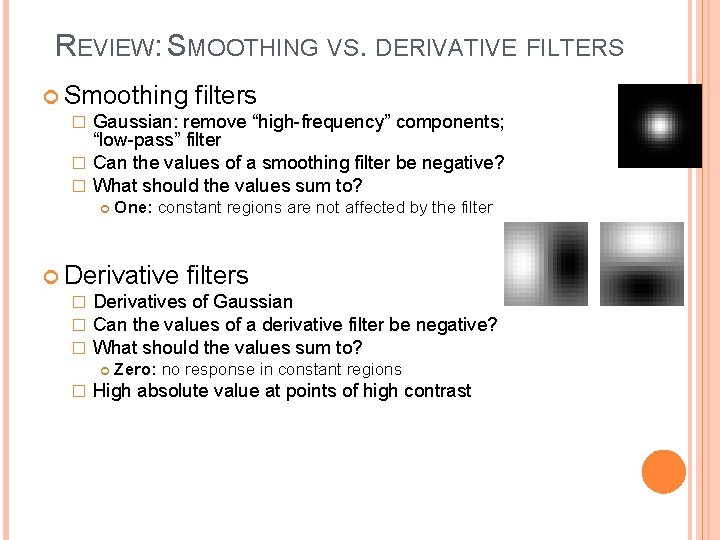REVIEW: SMOOTHING VS. DERIVATIVE FILTERS Smoothing filters � Gaussian: remove “high-frequency” components; “low-pass” filter � Can the values of a smoothing filter be negative? � What should the values sum to? One: constant regions are not affected by the filter Derivative filters � Derivatives of Gaussian � Can the values of a derivative filter be negative? � What should the values sum to? � Zero: no response in constant regions High absolute value at points of high contrast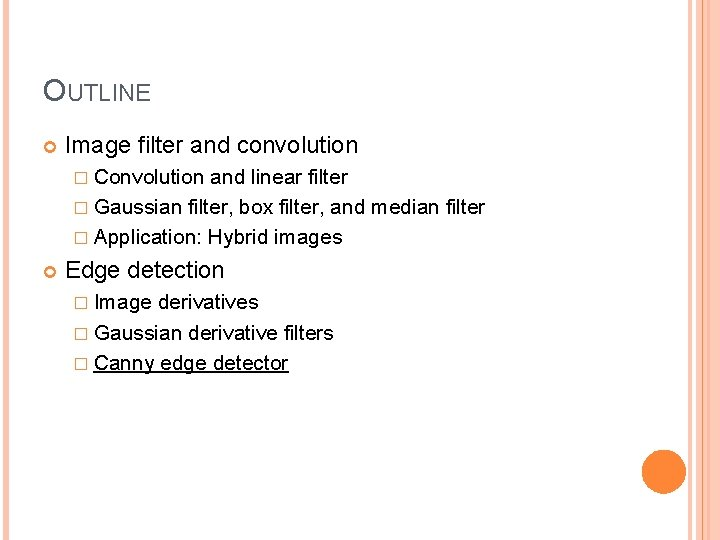OUTLINE Image filter and convolution � Convolution and linear filter � Gaussian filter, box filter, and median filter � Application: Hybrid images Edge detection � Image derivatives � Gaussian derivative filters � Canny edge detector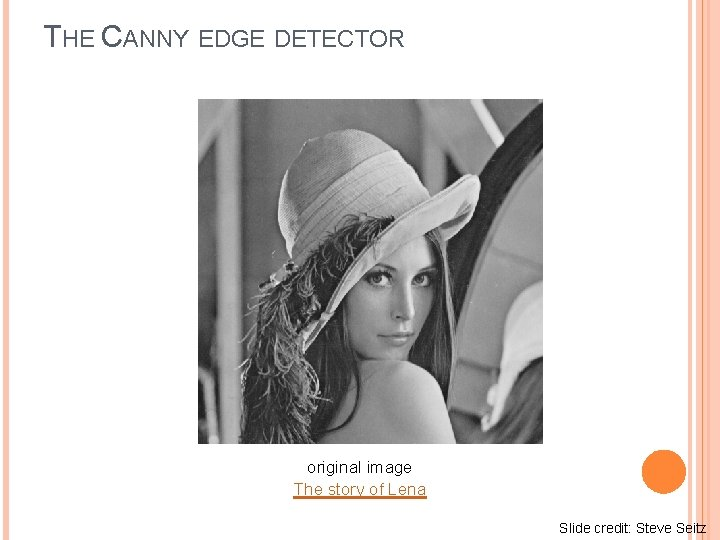THE CANNY EDGE DETECTOR original image The story of Lena Slide credit: Steve SeitzTHE CANNY EDGE DETECTOR norm of the gradient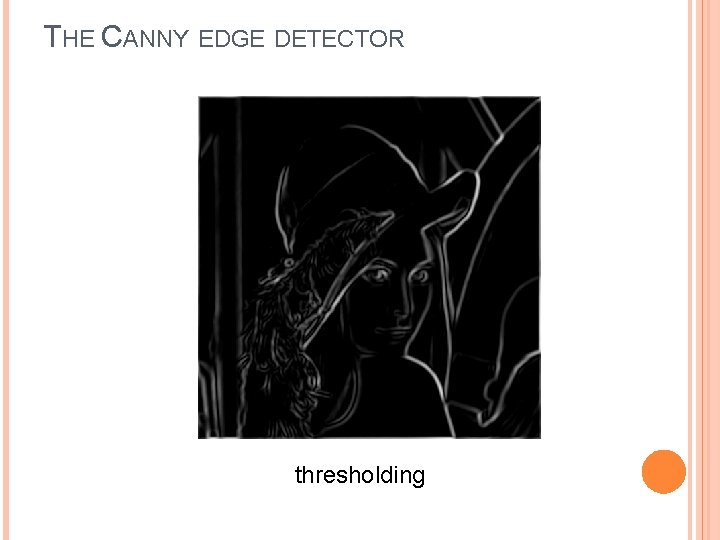THE CANNY EDGE DETECTOR thresholding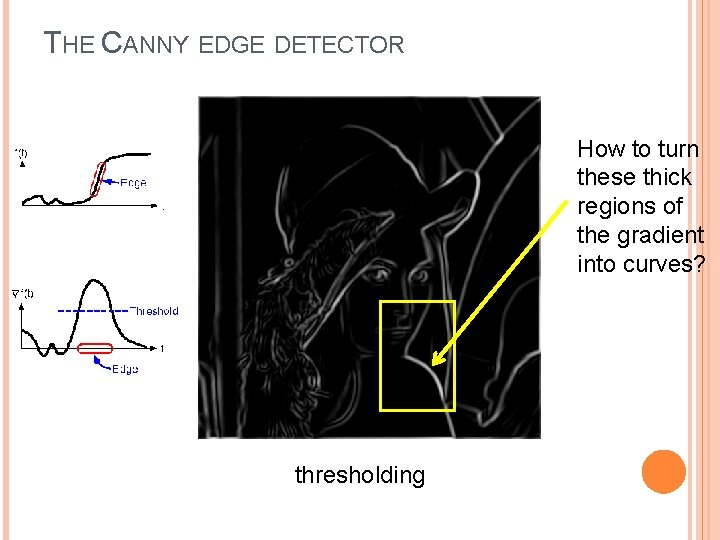THE CANNY EDGE DETECTOR How to turn these thick regions of the gradient into curves? thresholding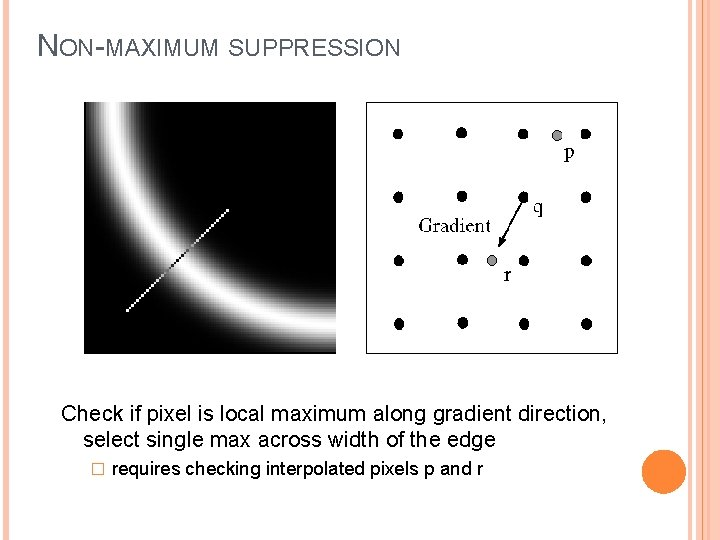NON-MAXIMUM SUPPRESSION Check if pixel is local maximum along gradient direction, select single max across width of the edge � requires checking interpolated pixels p and r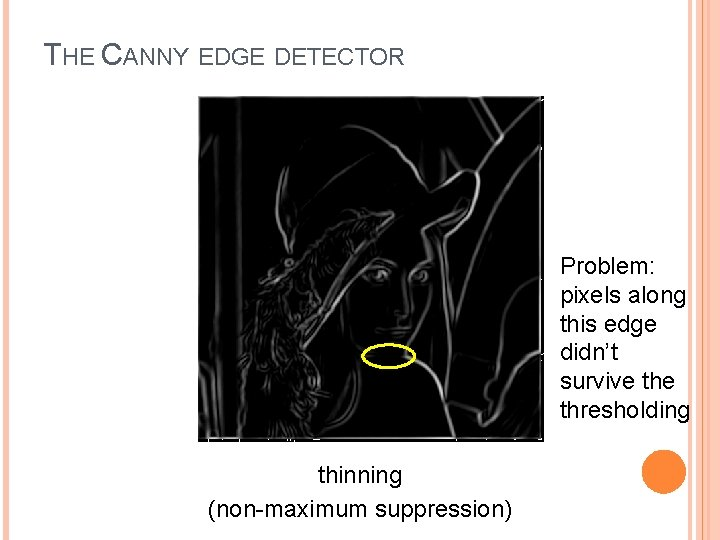THE CANNY EDGE DETECTOR Problem: pixels along this edge didn’t survive thresholding thinning (non-maximum suppression)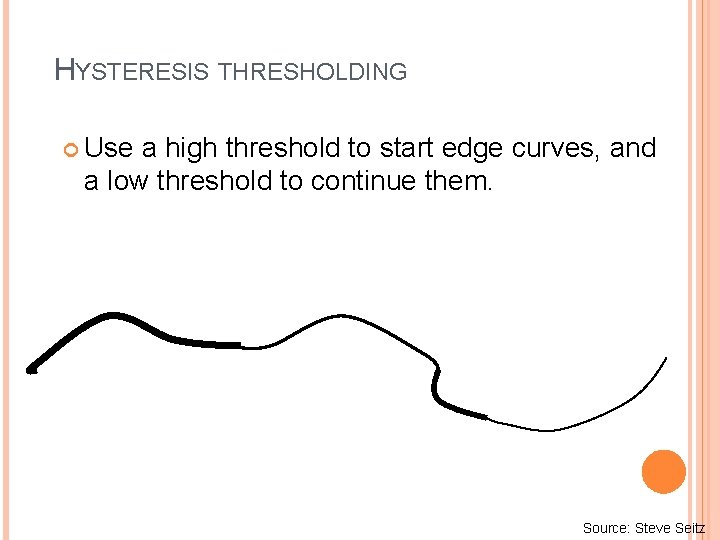HYSTERESIS THRESHOLDING Use a high threshold to start edge curves, and a low threshold to continue them. Source: Steve Seitz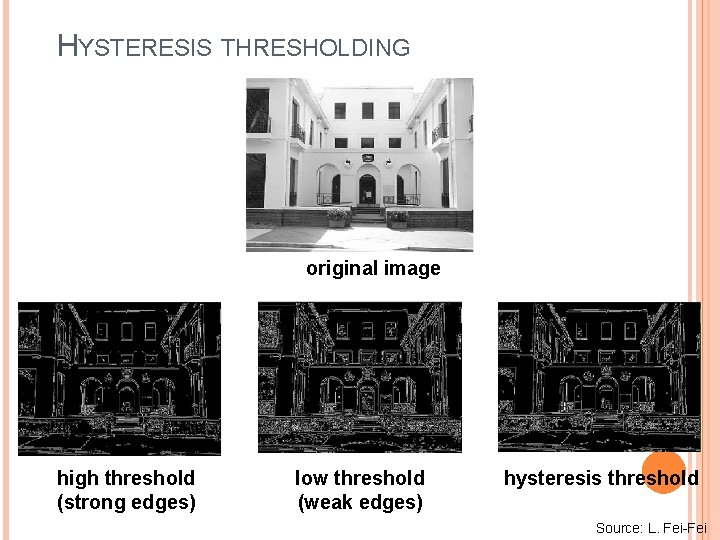HYSTERESIS THRESHOLDING original image high threshold (strong edges) low threshold (weak edges) hysteresis threshold Source: L. Fei-Fei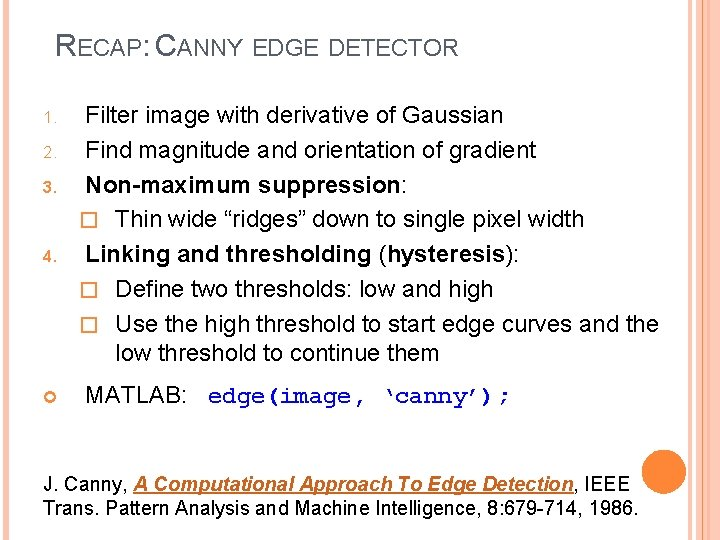RECAP: CANNY EDGE DETECTOR 1. 2. 3. 4. Filter image with derivative of Gaussian Find magnitude and orientation of gradient Non-maximum suppression: � Thin wide “ridges” down to single pixel width Linking and thresholding (hysteresis): � Define two thresholds: low and high � Use the high threshold to start edge curves and the low threshold to continue them MATLAB: edge(image, ‘canny’); J. Canny, A Computational Approach To Edge Detection, IEEE Trans. Pattern Analysis and Machine Intelligence, 8: 679 -714, 1986.EDGE DETECTION IS JUST THE BEGINNING… image human segmentation gradient magnitude Berkeley segmentation database: http: //www. eecs. berkeley. edu/Research/Projects/CS/vision/grouping/segbench/Low-level edges vs. perceived contours Background Kristen Grauman, UT-Austin Texture Shadows